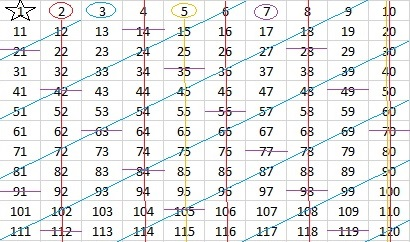# Finding Primes Like 1367

Ancient Greek Eratosthenes had a method of finding prime numbers. We call it the Sieve of Eratosthenes. You can use this method if you make a list of numbers, circle the first available prime number, cross out all of its multiples on the chart and repeat tat procedure over and over again. The next number that has not been crossed out is the next prime number. Numbers that get crossed out are composite numbers, and they can always be expressed as the product of prime numbers.

Many teachers have given their students a 100 chart to help them find the twenty-five prime numbers less than 100.If the number 1 were a prime number, we would have to cross out all of its multiples, and that would make 1 be the only prime number!?? That would be an unacceptable conclusion. It turns out that 1 cannot be either prime or composite. Perhaps you will want to put a star around it.

Here is a 100 chart with the multiples of 2, 3, 5, and 7 crossed out.Since 10 is a multiple of 2 and 5, it was easy to cross out their multiples. 9, a multiple of 3, is one less than 10, so 3’s multiples were also easy to cross out. Crossing out all the multiples of 7 is a little bit tedious at first, but you can even find a pattern for them as well.

Notice that the distance between multiples of any given prime is that prime number whether you count horizontally or vertically. The circled numbers AND all the numbers not crossed out on the chart are prime numbers.

This Sieve of Eratosthenes is a powerful method for finding prime numbers, but some of its power is lost when just a 100 chart is used. For pretty much the same amount of work, we could have used the list of numbers in a 10 × 12 chart because 10 × 12 = 120 which is one less than the next prime number squared, 11² = 121.But wait a minute! 11 is one more than 10 so its multiples would be SO easy to cross out. Let’s make the chart go to one less than 168 which is one less than 13²:Yeah, it’s not a perfect rectangle anymore, but for about the same effort, we get an additional fourteen prime numbers. I also like that all of these multiples of seven {21, 42, 63, 84, 105, 126, 147, 168} are sort of on a diagonal. What do you notice about the numbers in that set? The next number in the set isn’t on the chart, but can you figure out what it is and where it goes?

To find out if a number like 1367 is prime, I wouldn’t want to expand a 100 chart into a 1400 chart. That chart would also require me to cross out all the multiples of every prime number from 13 to 31, and that might be a big pain.  It’s much easier to see if 1367 is divisible by any of the prime numbers less than its square root. It isn’t, so I conclude:

• 1367 is a prime number.
• Prime factorization: 1367 is prime.
• 1367 has no exponents greater than 1 in its prime factorization, so √1367 cannot be simplified.
• The exponent in the prime factorization is 1. Adding one to that exponent we get (1 + 1) = 2. Therefore 1367 has exactly 2 factors.
• The factors of 1367 are outlined with their factor pair partners in the graphic below.How do we know that 1367 is a prime number? If 1367 were not a prime number, then it would be divisible by at least one prime number less than or equal to √1367. Since 1367 cannot be divided evenly by 2, 3, 5, 7, 11, 13, 17, 19, 23, 29 or 31, we know that 1367 is a prime number.

This site uses Akismet to reduce spam. Learn how your comment data is processed.